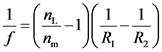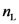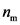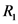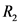# What is the focal length f of this lens in air (index of refraction for air is nair=1)?

A “biconvex” lens is one in which both surfaces of the lens
bulge outwards. Suppose you had a biconvex lens with radii of
curvature with magnitudes of |R1|=10cm and
|R2|=15cm. The lens is made of glass with index of
refraction nglass=1.5. We will employ the convention that
R1 refers to the radius of curvature of the surface
through which light will enter the lens, and R2 refers to
the radius of curvature of the surface from which light will exit
the lens. R R n R R C) What is the focal length of the lens if it is immersed in
water (nwater = 1.3)
f= ____________ cm water What is the focal length f of this lens in air (index
of refraction for air is nair=1)?
f n

General guidance

Concepts and reason
The concept used in this question is lens maker formula.
Firstly, write the expression of the lens maker formula and use it to find the focal length of the lens when it is immersed in water.
Finally, use the formula to find the focal length of the lens when it is placed in air.

Fundamentals

According to the thin lens formula, the focal length of the lens when it is placed in a medium can be determined by the following expression:

Here, f is the focal length of the lens, is the refractive index of the lens, is the refractive index of the medium, , and are the radius of curvature of the lens through which the

Step-by-step

Step 1 of 2

(a)
The lens maker formula is as follows:

The lens is immersed in water so that the medium is water. The radius of curvature of the two curved surfaces of the lens are as follows:

Substitute 1.5 for , 1.3 for , 10 cm for , and -15 cm for in the above expression:

Part a
The focal length is 39 cm.

The lens maker’s formula is a relation that connects the radii of curvature of the two surfaces of a lens with the focal length and the refractive index of the lens and the refractive index of the medium in which the lens is kept.

Use the formula to find the focal length of the lens when it is placed in air.

Step 2 of 2

(b)
The lens maker formula is as follows:

The lens is placed in air so that the medium is air. The radius of curvature of the two curved surfaces of the lens are as follows:

Substitute 1.5 for , 1.0 for , 10 cm for , and -15 cm for in the above expression:

Part b
The focal length is 12 cm.

The formula uses the assumptions that the lens is thin with a small aperture. The incident and the refracted rays makes small angles with each other.

Do not use the radius of curvature to be positive in both cases. The radius measured in the direction of the incident rays are considered to be positive and the radius against the direction of incident rays are considered to be negative. Here, is positive and is negative.

Part a
The focal length is 39 cm.

Part b
The focal length is 12 cm.

Part a
The focal length is 39 cm.

Part b
The focal length is 12 cm.

(?)();
(?)();
R, = 10 cm R, =-15 cm
-13) 10cm -15 cm) = (0.153846)(0.1+0.0666666) cm = 0.025641 cm f = 39 cm
(?)();
R, = 10 cm R, =-15 cm
(15-1_1_ 1 ilio-‘|10 cm -15 cm) = (0.5)(0.1+0.0666666) cm =0.083333 cm f =12 cm
We were unable to transcribe this image